Function Repository Resource:

# PairwiseMultidimensionalScaling

Multidimensional scaling algorithm for embedding pairwise distances into a Cartesian space

Contributed by: Nikolay Murzin
 ResourceFunction["PairwiseMultidimensionalScaling"][dm] return a list of 2-dimensional coordinates representing embedding of a distance matrix dm. ResourceFunction["PairwiseMultidimensionalScaling"][dm,dim] embed into a given integer dimension dim.

## Details

Usually the multidimensional scaling algorithm is used for a given list of vectors, and there is an intermediate step of computing distances between them. In some cases, only the distances are known and the task is to find a lower-dimensional embedding such that the resulting distances are approximately preserved.
DimensionReduce with Method "MultidimensionalScaling"or ResourceFunction["MultidimensionalScaling"] can be used for computing Multidimensional scaling for a list of vectors.
ResourceFunction["PairwiseMultidimensionalScaling"] implements classical multidimensional scaling, which is an exact solution to a minimization of the strain function problem.

## Examples

### Basic Examples (2)

Compute 2-dimensional embedding from a distance matrix of three points:

 In:=Out=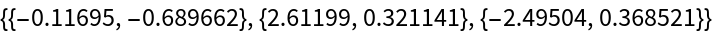Compute 3-dimensional embedding from a distance matrix of four points:

 In:=Out=### Applications (3)

Coordinatize an edge-weighted graph:

 In:=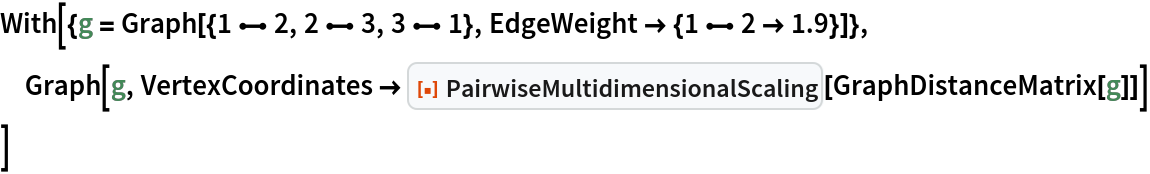Out=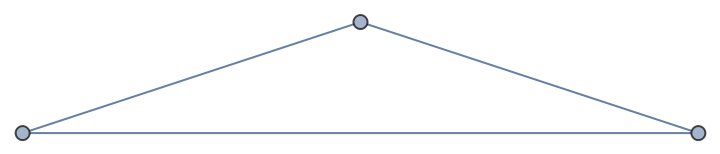Coordinatize a graph given pairwise distances between vertices:

 In:=Out=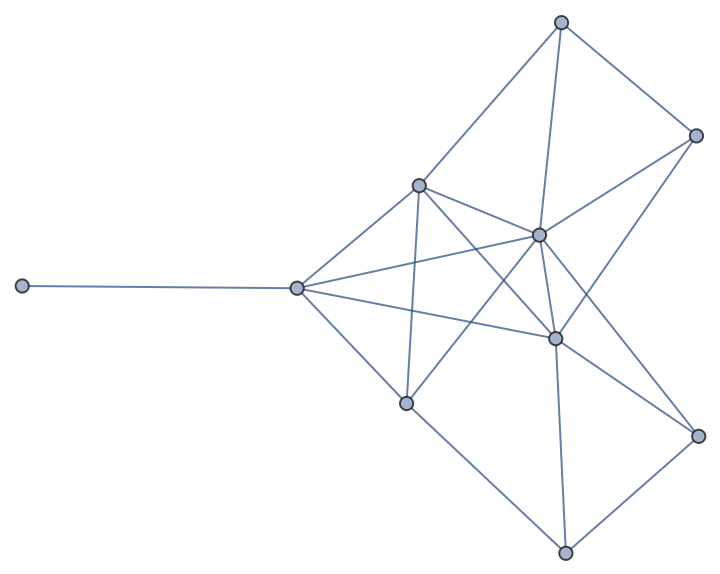Providing distances of its GraphEmbedding the original graph can be closely reconstructed (up-to translational/rotational/reflectional symmetry):

 In:=Out=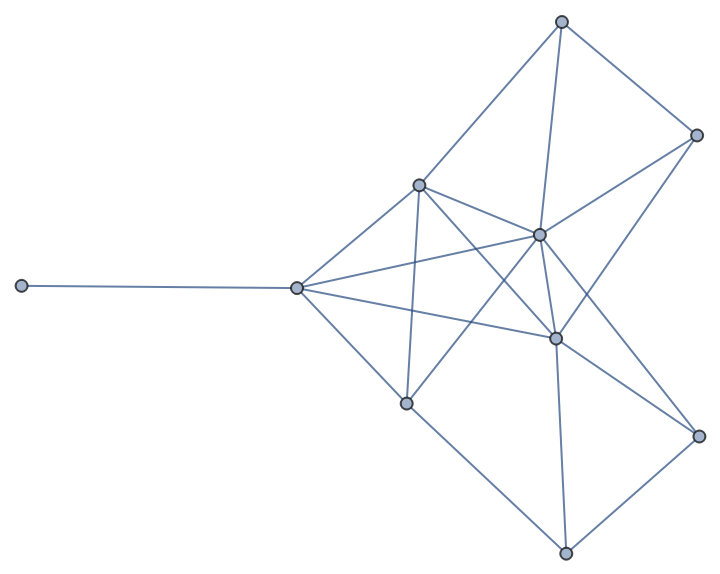Coordinatize a causal graph:

 In:=Out=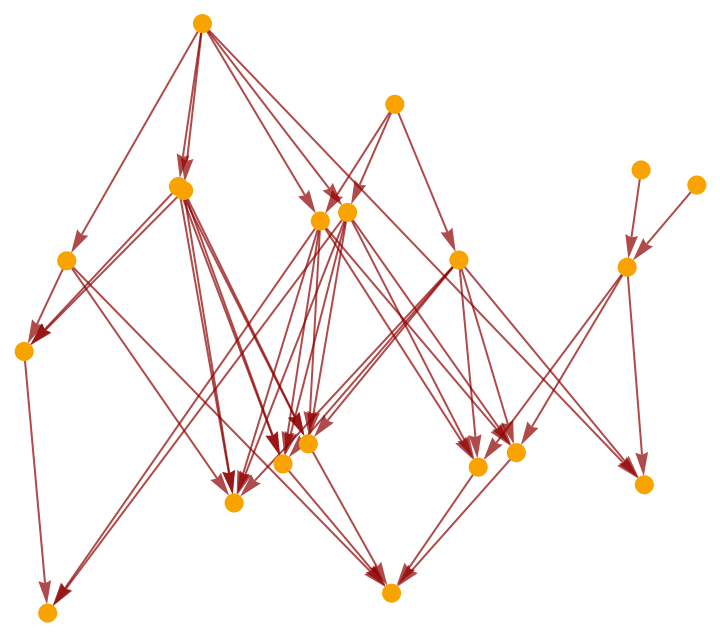In:=Out=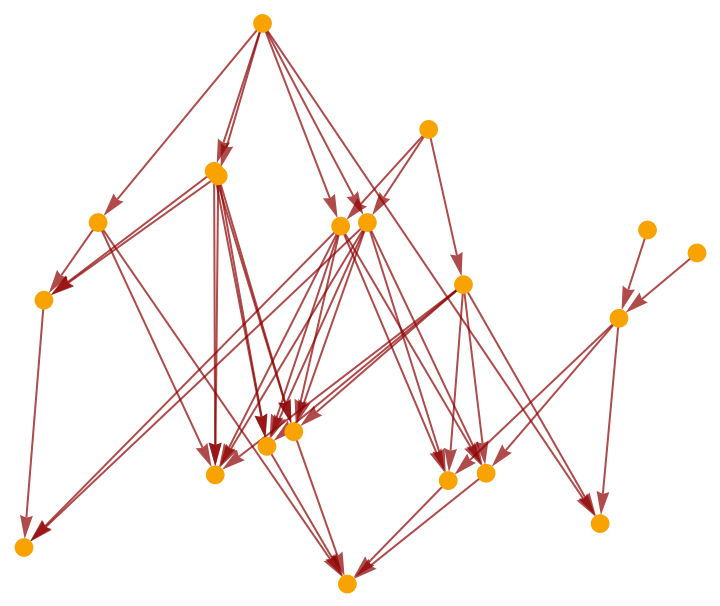#### Properties and Relations (1)

PairwiseMultidimensionalScaling embedding is homomorphic to the one computed using ResourceFunction[“MultidimensionalScaling”] for a DistanceMatrix with DistanceFunction EuclideanDistance, and also similar to DimensionReduce with various methods:

 In:=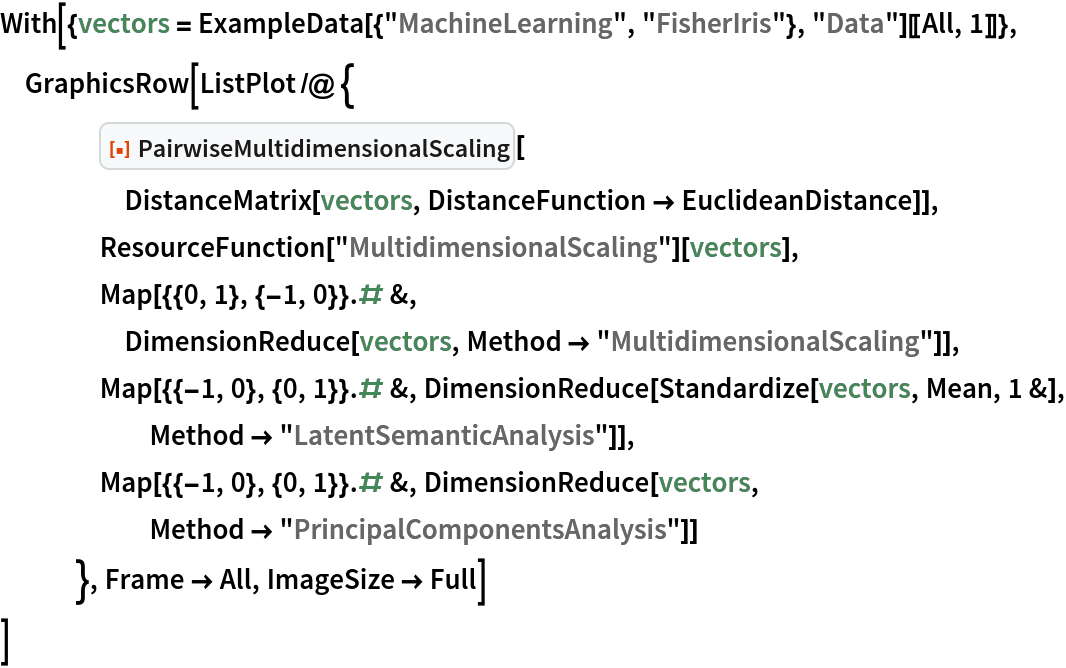Out=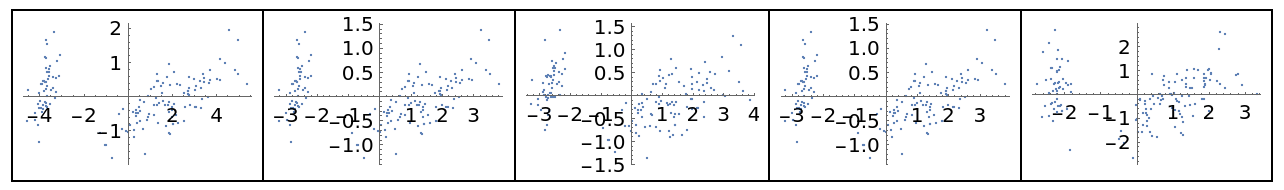## Version History

• 1.0.0 – 28 September 2022